Calculating BMI – another GUI in Matlab

In this project, we are going to develop a GUI in Matlab to calculate the BMI (body mass index) of any people. We are going to introduce the radio button, which we haven’t explored before in this site, and we’re going to include a button to save the data in an external spreadsheet. We could use these concepts to develop complex databases, but we are going to keep things very simple in this case.

If you do not want to create a GUI but to use an online calculator, go here.

If you haven’t read the main concepts of GUI development, I suggest you read them before you continue with this experiment. You can find here the basics of GUI development, and here the basics of callbacks. It’s important that you understand those concepts first.

Note: the BMI calculator that we’re going to develop here is like an experiment with a scientific toy, and it’s not intended for serious purposes. Use at your own risk.

Open the guide tool in Matlab and choose the blank GUI option.

Then, replicate this screen and save your project as bmi.fig.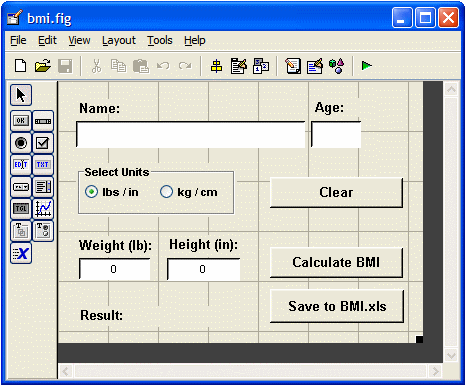The window is going to have four edit boxes (or objects) to be used as name, age, weight and height inputs. The name and age are just for documentation purposes, and obviously they don’t matter at all for the calculation process. Each edit box has an informative associated label. The labels can be obtained with text objects.

This GUI is going to have a group of two radio buttons (within a panel object) to let the user select the units (anglo or metric), and it’s going to have three buttons, one to reset or clear the input, another to perform the calculations and, the third button for saving the information in a separate file, in this case it’ll be an Excel spreadsheet.

These are the components utilized in my GUI. Blank spaces mean the default value provided by Matlab: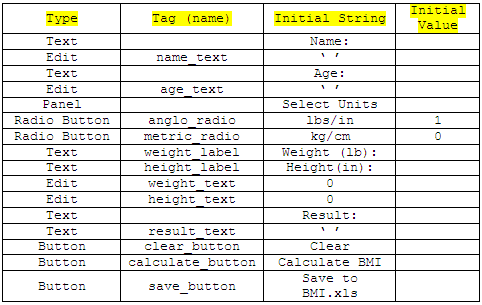The tag names are associated with the functions generated by the guide tool, that’s why it’s important to follow them. The initial strings and values are the initial conditions of the GUI and that’s what the user sees when executing the script.

Initial Conditions

When you save the bmi.fig file with the objects drawn and named as detailed above, Matlab creates a kind of template. You have to “fill-in the blanks” to make it work...

Almost at the top of your new template, you’ll see something like this function, but with less lines. Make sure that at the end, you have all these lines...

% --- Executes just before bmi is made visible.
function bmi_OpeningFcn(hObject, eventdata, handles, varargin)
% This function has no output args, see OutputFcn.
% hObject    handle to figure
% eventdata reserved - to be defined in a future version of MATLAB
% handles structure with handles and user data (see GUIDATA)
% varargin command line arguments to bmi (see VARARGIN)
% Choose default command line output for bmi

handles.output = hObject;

handles.metric = 0;
handles.wu =
'lb';
handles.hu =
'in';
handles.name =
'';
handles.age =
'';
handles.bmi = 0;
handles.weight =
'';
handles.height =
'';
handles.result =
'';
handles.condition =
'';

% Update handles structure
guidata(hObject, handles);

These variables are necessary if the user doesn’t use the GUI as intended. If the user doesn’t enter data as expected and we don’t use the initial conditions, the GUI doesn’t behave correctly and crashes. The conditions above are useful to give stability to our design.

Entering Name and Age

I’m going to mention only the changes that you have to make to your template. The auto-generated template contains many comments. Leave them in your code if you want, but I won’t copy all those comments here. Use the name of the functions to follow and make the relevant modifications.

% --- Executes on text input in name_text.
function name_text_Callback(hObject, eventdata, handles)
handles.name = get(hObject,
'String');
guidata(hObject, handles)

% --- Executes on text input in age_text.
function age_text_Callback(hObject, eventdata, handles)
handles.age = get(hObject,
'String');
guidata(hObject, handles)

Selecting Units

% --- Executes on button press in anglo_radio.
handles.anglo = get(hObject,
'Value');
set(handles.weight_label,
'String', 'Weight (lb):')
set(handles.height_label,
'String', 'Height (in):')
'Value', 0)
handles.metric = 0;
handles.wu =
'lb';
handles.hu =
'in';
guidata(hObject, handles)

% --- Executes on button press in metric_radio.

handles.metric = get(hObject,
'Value');
set(handles.weight_label,
'String', 'Weight (kg):')
set(handles.height_label,
'String', 'Height (cm):')
'Value', 0)
handles.anglo = 0;
handles.wu =
'kg';
handles.hu =
'cm';
guidata(hObject, handles)

Entering Weight and Height

% --- Executes on text input in weight_text.
function weight_text_Callback(hObject, eventdata, handles)
num = str2double(get(hObject,
'String'));
if isnan(num)
set(hObject,
'String', 0);
end
handles.weight = num;
guidata(hObject, handles)

% --- Executes on text input in height_text.
function height_text_Callback(hObject, eventdata, handles)
num = str2double(get(hObject,
'String'));
if isnan(num)
set(hObject,
'String', 0);
end
handles.height = num;
guidata(hObject, handles);

Calulating BMI

The formula for the BMI calculation is

BMI = 104 x (weight in kg) / (height in cm)2

if you want to use pounds and inches instead, the formula is

BMI = 104 x (weight in lbs/2.2046) / (height in in/0.3937)2

The table to determine the condition is

BMI < 18.5 Underweight
18.5 – 24.9 Normal
25   – 29.9 Overweight
> 30   Obese

The formula to calculate BMI and the code to look-up the table can be coded as follows:

% --- Executes on button press in calculate_button.
function calculate_button_Callback(hObject, eventdata, handles)
if handles.metric
w = handles.weight;
h = handles.height;

else
w = handles.weight/2.2046;
h = handles.height/0.3937;

end

bmi = 1e4 * w/h^2;

if bmi < 18.5
s =
' Underweight';
elseif 18.5 <= bmi & bmi < 25
s =
' Normal';
elseif 25 <= bmi & bmi < 30
s =
' Overweight';
else
s = ' Obese';
end

bmis = [num2str(bmi, 3) s];
set(handles.result_text,
'String', bmis);
handles.result = bmi;
handles.condition = s;
guidata(hObject,handles);

Clearing the Graphic Interface

% --- Executes on button press in clear_button.
function clear_button_Callback(hObject, eventdata, handles)
set(handles.name_text,
'String', '')
handles.name =
'';
set(handles.age_text,
'String', '')
handles.age =
'';
set(handles.weight_text,
'String', '0')
handles.weight = 0;
set(handles.height_text,
'String', '0')
handles.height = 0;
set(handles.result_text,
'String', '')
handles.result = 0;
handles.condition =
'';

Saving the data in an Excel file

This process can be done in many ways. In this case we’re going to keep things simple and use just the functions xlsread and xlswrite. With xlsread we’re going to read the number of lines of information (registers) that the file has, and we’re using xlswrite to write one more line (register of eight columns or fields) to the file. Here, you can read a full article about data interchange between Matlab and Excel.

Beforehand, we’re going to prepare a spreadsheet named bmi.xls, and we’re going to save it in the same working directory that we’re using with Matlab. A file like this: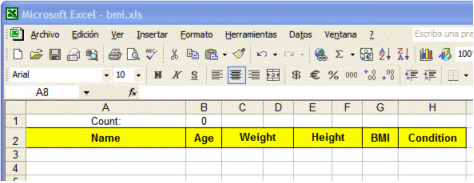We have eight columns, for all the information that our graphical interface is going to produce. In cell B1, we have this formula: +COUNT( G3 : G65536 ). It just counts all the registers that we have in the spreadsheet. We can later hide that line (row 1) to avoid distractions.

At the beginning, the count is 0 (as shown), of course. That means that we could add a register in line 3, which is the first line available. If we had a count of 5, we could add a register in  the 8th line. The count is read with Matlab built-in function xlsread. Then, we prepare the 8-field register in a cell-array in Matlab and save it tho the sheet 1 of the file ‘bmi.xls’.

We can even think of a pop-up window to display success or failure of the saving process...

That’s accomplished with this code:

% --- Executes on button press in save_button.
function save_button_Callback(hObject, eventdata, handles)
d{1, 1} = handles.name;
d{1, 2} = handles.age;
d{1, 3} = handles.weight;
d{1, 4} = handles.wu;
d{1, 5} = handles.height;
d{1, 6} = handles.hu;
d{1, 7} = handles.result;
d{1, 8} = handles.condition;

c = xlsread('bmi', 1 , 'b1');
position = [
'a' num2str(c+3)];
[status, message] = xlswrite(
'bmi', d, 1, position);

if status
helpdlg(
else
errordlg('Could not save data', 'Save Spreadsheet');
end

These are some screenshots...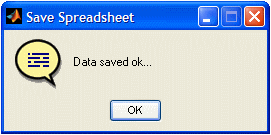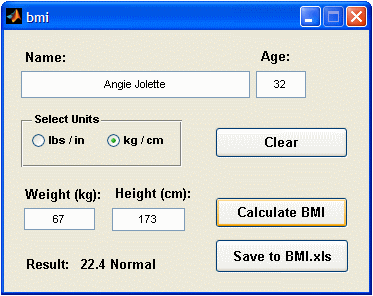So the project seems to be working fine!

From 'Calculating BMI' to Matlab home

From 'Calculationg BMI' to Matlab GUIs

 Top